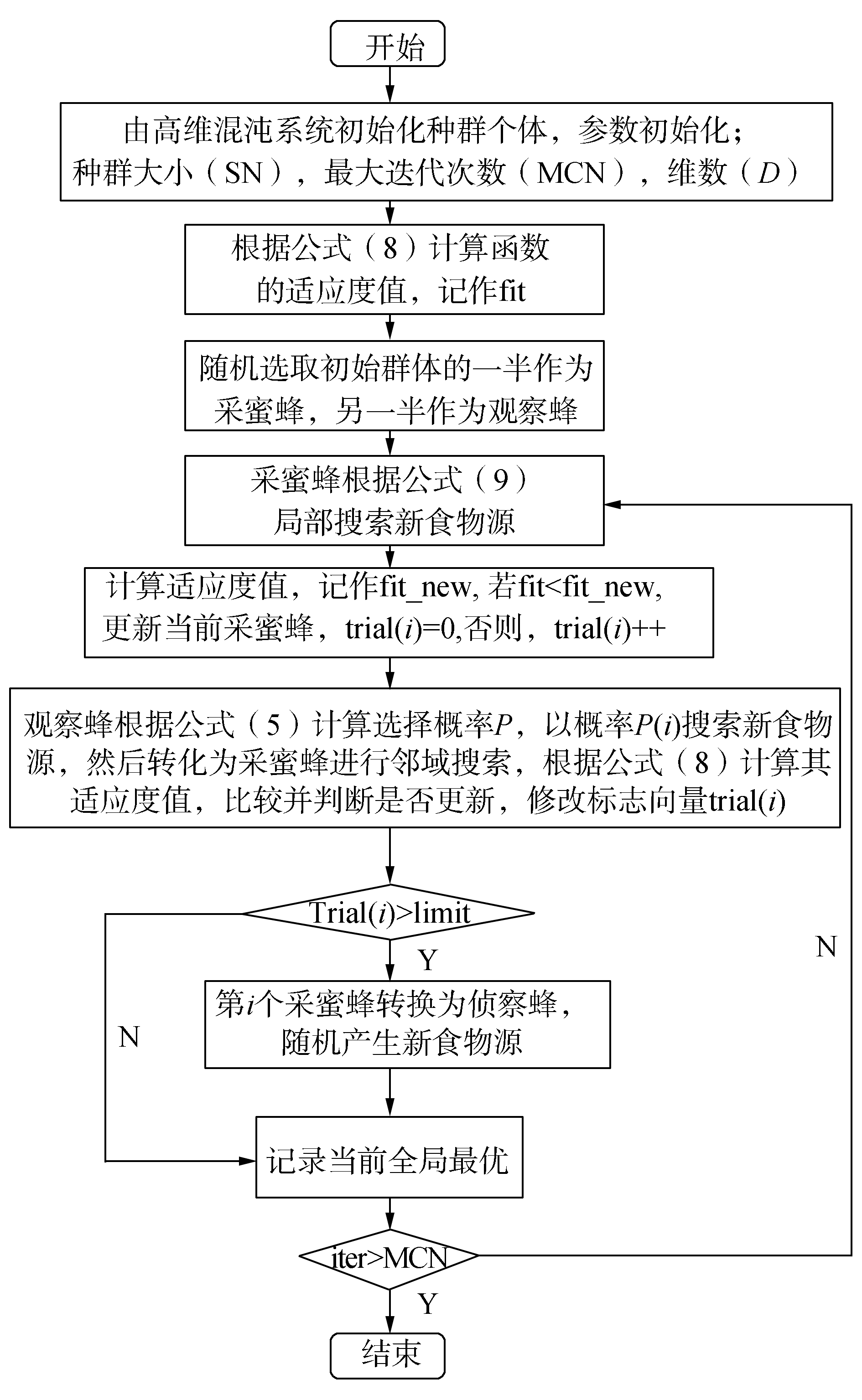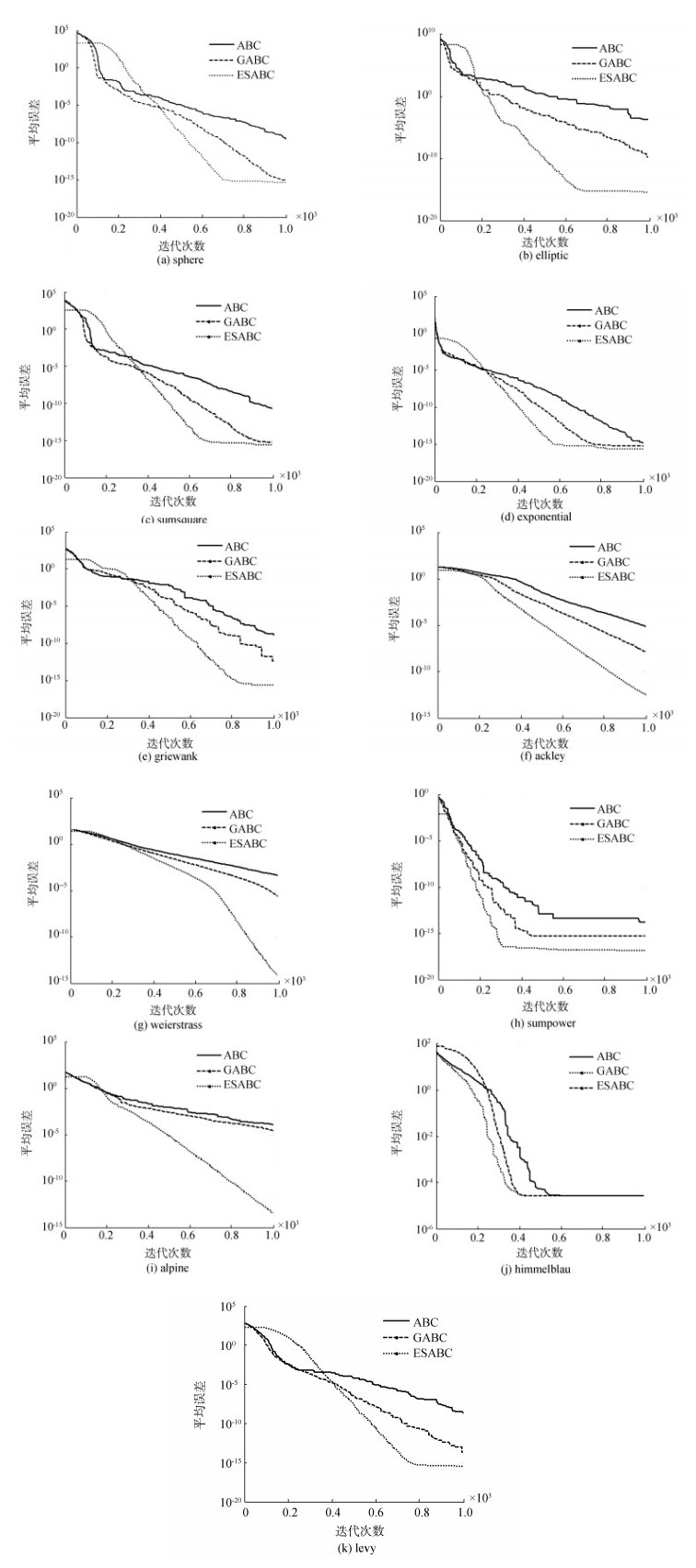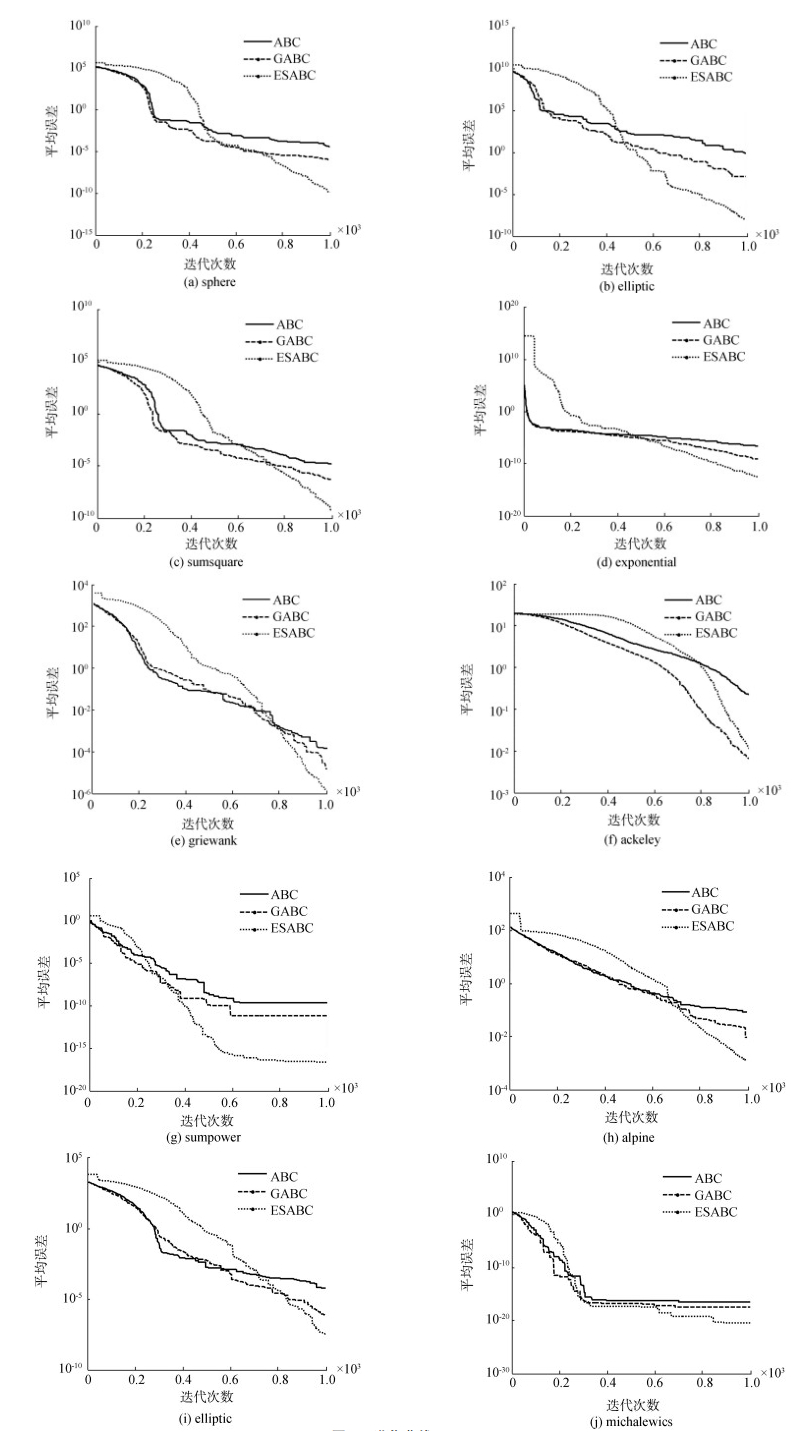﻿ 一种增强局部搜索能力的改进人工蜂群算法
«上一篇文章快速检索 高级检索

 智能系统学报2017, Vol. 12Issue (5): 684-693  DOI: 10.11992/tis.2016120260

### 引用本文LIU Xiaofang, LIU Peizhong, LUO Yanmin, et al. Improved artificial bee colony algorithm based on enhanced local search[J]. CAAI Transactions on Intelligent Systems, 2017, 12(5): 684-693. DOI: 10.11992/tis.201612026.### 文章历史

1. 华侨大学 工学院, 福建 泉州 362021;
2. 华侨大学 计算机科学与技术学院, 福建 厦门 361021

Improved artificial bee colony algorithm based on enhanced local search
LIU Xiaofang1, LIU Peizhong1, LUO Yanmin2, FAN Yuling1
1. Engineering school, Huaqiao University, Quanzhou 362021, China;
2. School of Computer Science and Technology, Huaqiao University, Xiamen 361021, China
Abstract: The shortcomings of the artificial bee colony algorithm (ABC) are its uneven initial population distribution and weak local search. In this paper, we propose an ABC algorithm based on enhanced local search (ESABC). First, we employ a high-dimension chaotic system (Lorenz system) to obtain the ergodic and regular initial populations and to avoid the blindness of random initialization in the population initialization stage. Then, we introduce improved fitness evaluation methods based on the logarithmic function to increase the differences between individuals, reduce selection pressure, and avoid premature convergence. Lastly, inspired by the differential evolution algorithm, we propose a new search tactic that uses the best individual in the contemporary population to guide the renewal of the next generation, and thereby enhance the local search ability. We examined the performance of the proposed approach with 12 classic testing functions and compared the results with the basic and other ABCs. As documented in the experimental results, the proposed algorithm exhibits good optimization performance and can improve both the accuracy and convergence speed of the algorithm.
Key words: artificial bee colony algorithm    high-dimension chaotic system    fitness evaluation    search tactics    optimization algorithm    evolutionary algorithm    convergence analysis    accuracy analysis    intelligent algorithm

1 基本的人工蜂群算法

 ${x_i}^j = {x_{{\rm{min}}}}^j + {\rm{rand}}\left( {0, 1} \right)({x_{{\rm{max}}}}^j-{x_{{\rm{min}}}}^j)$ (1)

 ${v_i}^j = {x_i}^j + {\varphi _i}^j({x_i}^j-{x_k}^j)$ (2)

 ${\rm{fi}}{{\rm{t}}_i} = \left\{ \begin{array}{l} 1/(1 + {f_i}), {\rm{ }}{f_i} \ge 0\\ 1 + {\rm{abs}}({f_i}), {\rm{ }}{f_i} \le 0 \end{array} \right.{\rm{ }}$ (3)

 $P\{ {T_s}({X_i}, {V_i}) = {V_i}\} = \left\{ \begin{array}{l} 1, {\rm{ }}f({V_i}) \ge f({X_i})\\ 0, {\rm{ }}f({V_i}) < f({X_i}) \end{array} \right.{\rm{ }}$ (4)

 ${p_i} = \frac{{{\rm{fi}}{{\rm{t}}_i}}}{{\sum\limits_{i = 1}^{{\rm{SN}}} {{\rm{fi}}{{\rm{t}}_i}} }}$ (5)

2 改进的人工蜂群算法

2.1 种群初始化

 $\left\{ \begin{array}{l} \dot x = \delta \left( {y-x} \right)\\ \dot y = \gamma x-y-xz\\ \dot z = xy - \beta z \end{array} \right.$ (6)

 ${x_i}^j = {x_{{\rm{min}}}}^j + \varphi ({x_{{\rm{max}}}}^j-{x_{{\rm{min}}}}^j)$ (7)

2.2 改进的适应度评价方式

 ${\rm{fi}}{{\rm{t}}_i} = 0.1/(0.1 + 1/\left| {{\rm{lg}}\;{f_i}} \right|), {\rm{ }}0 \le {f_i} \le {10^{-\lambda }}$ (8)

2.3 新的搜索机制

 ${v_i}^j = {x_{{\rm{best}}}}^j + {\phi _i}^j({x_{{\rm{best}}}}^j-{x_r}^j)$ (9)

2.4 改进算法流程图图 1 ESABC算法流程图 Fig.1 ESABC flowchat
3 实验结果与分析 3.1 测试函数表 1 基准函数 Tab.1 Benchmark Functions
3.2 实验分析表 2 实验结果(D=30) Tab.2 Experiential results(D=30)表 3 实验结果(D=60) Tab.3 Experiential results(D=60)图 2 进化曲线(D=30) Fig.2 Evolution curves(D=30)图 3 进化曲线(D=60) Fig.3 Evolution curves(D=60)

4 结束语

  KARABOGA D. An idea based on honey bee swarm for numerical optimization. technical report-TR06[R]. Kayseri:Erciyes University, 2005. (0)  KARABOGA D, BASTURK B. On the performance of artificial bee colony (ABC) algorithm[J]. Applied soft computing, 2008, 8(1): 687-697. DOI:10.1016/j.asoc.2007.05.007 (0)  KARABOGA D, AKAY B. A comparative study of artificial bee colony algorithm[J]. Applied mathematics and computation, 2009, 214(1): 108-132. DOI:10.1016/j.amc.2009.03.090 (0)  秦全德, 程适, 李丽, 等. 人工蜂群算法研究综述[J]. 智能系统学报, 2014, 9(2): 127-135. QIN Quande, CHENG Shi, LI Li, et al. Artificial bee colony algorithm:a survey[J]. CAAI transactions on intelligent systems, 2014, 9(2): 127-135. DOI:10.3969/j.issn.1673-4785.201307019 (0)  ZHU G, KWONG S. Gbest-guided artificial bee colony algorithm for numerical function optimization[J]. Applied mathematics & computation, 2010, 217(7): 3166-3173. (0)  姜建国, 叶华, 刘慧敏, 等. 融合快速信息交流和局部搜索的粒子群算法[J]. 哈尔滨工程大学学报, 2015, 36(5): 687-691. JIANG Jianguo, YE Hua, LIU Huimin, et al. Particle swarm optimization method with combination of rapid information communication and local search[J]. Journal of Harbin engineering university, 2015, 36(5): 687-691. (0)  GAO Weifeng, Liu Sanyang, et al. Improved artificial bee colony algorithm for global optimization[J]. Information processing letters, 2011, 111(17): 871-882. DOI:10.1016/j.ipl.2011.06.002 (0)  GAO Weifeng, LIU Sanyang. A modified artificial bee colony algorithm[J]. Computers and operations research, 2012, 39(3): 687-697. DOI:10.1016/j.cor.2011.06.007 (0)  GAO W F, LIU S Y, HUANG L L. A novel artificial bee colony algorithm with method[J]. Applied soft computing, 2013, 13(9): 3763-3775. DOI:10.1016/j.asoc.2013.05.012 (0)  GAO W F, LIU S Y, HUANG L L. Enhancing artificial bee colony algorithm using more information-based search equations[J]. Information sciences, 2014, 270(1): 112-133. (0)  GAO W, CHAN F T S, HUANG L, et al. Bare bones artificial bee colony algorithm with parameter adaptation and fitness-based neighborhood[J]. Information sciences, 2015, 316(C): 180-200. (0)  ALATAS B. Chaotic bee colony algorithms for global numerical optimization[J]. Expert systems with applications, 2010, 37(8): 5682-5687. DOI:10.1016/j.eswa.2010.02.042 (0)  陈杰, 沈艳霞, 陆欣. 基于信息反馈和改进适应度评价的人工蜂群算法[J]. 智能系统学报, 2016, 11(2): 172-179. CHEN Jie, SHEN Yanxia, LU Xin. Artificial bee colony algorithm based on information feedback and an improved fitness value evaluation[J]. CAAI transactions on intelligent systems, 2016, 11(2): 172-179. (0)  YI, Wenchao, et al. Differential evolution algorithm with variable neighborhood search for hybrid flow shop scheduling problem[C]//IEEE, International Conference on Computer Supported Cooperative Work in Design IEEE. Nanchang, China 2016:233-238. (0)  KIRAN M S, HAKLI H, GUNDUZ M, et al. Artificial bee colony algorithm with variable search strategy for continuous optimization[J]. Information sciences, 2015, 300: 140-157. DOI:10.1016/j.ins.2014.12.043 (0)  SUGANTHAN P N, HANSEN N, LIANG J J, et al. Problem definitions and evaluation criteria for the CEC 2005 special session on real-parameter optimization[R]. KanGAL Report #2005005. India:ⅡT Kanpur, 2005. (0)  王志刚, 王明刚. 基于符号函数的多搜索策略人工蜂群算法[J]. 控制与决策, 2016, 31(11): 2037-2044. WANG Zhigang, WANG Minggang. Multi-search strategy of artificial bee colony algorithm based on symbolic function[J]. Control and decision, 2016, 31(11): 2037-2044. (0)